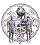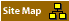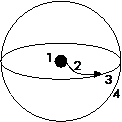## Hi, Walter, Jon, Jos, Peter, Goro, Michael, Karl,Nassim, Brian, Cyril, Diego, Daniel, Edgar, Elizabeth, Gilles, Jerry, Joachim, Kathleen, Stuart, Roel

### How to mathematically describe a universal point?

Today I write to ask you:
Can you help?

Ever since I started to get to know mathematics, in engineering school, I sought solution for the following image:Figure 1. Creation of a mathematical/mental/realisational/observational 4D Space

1. logical operations; =, ≠, ≈, ≤/≥, … distinction/identification: Freedom of Choice
2. mathematical operators: +/-/*/÷; …
3. mathematical functions; , x, ℓ/e, φ, π, …
4. values: 0, 1, N, ∞, …

The image represents the transition from a point via a spiral to a circle which then spins around on itself to a Form.
It is the basis for the development of any/every mathematical reference system.
It links scalar (0D, ‘black hole’) to vector (Catastrophe) to array (Field) to a holon (3D Soliton).
It is the foundation for the organisation of atoms (“neutron, proton, electron, photon”), thus matter.

Starting point is a splitting in/of the point in the centre; this is a Logic operation.
Second step is an extension, which is equivalent to any mathematical Operator.
Third is the formulation of a definition, which is a mathematical Function/equation.
Finally it results in a defined form; which is equivalent to a state or Value.

The first step involves our involvement; it is a dimensional operation (choice/decision).
The second stage is that of continuation, which is a form of participation.
The third phase is that of definition, which is a form of evaluation.
The final fourth act is a validation; systemic and observational/creational closure.

Do you know any mathematical form, or form of mathematics, which can describe this?
If not, do you know any mathematical form or function which can be used towards this?
As you will understand: logic, operation, function and value are now one operation.
It is the operation of the mathematician defining mathematics: consciousness.

The figure is a development from Point to Line to Plane to Volume.
That means that it links 0D to 1D to 2D to 3D: it is a 4D Vortex.
Therein any of the operations is complete in itself; the Logic, Operation, Function and Value.
It means that this schema is the representation of our core consciousness function; and the basis of life.

What I seek is … a way to describe it; ‘some mathematical formulation’ for this.
I know that this schema integrates/unifies all forms of mathematics.
It thereby likewise unifies/integrates all the forms of science.
But those formulations lack the description of our involvement.

What this schema needs is a formulation for the logic in/as/of participation in creation.
Ever since is ‘saw’ it, i have been able to work with it mentally.
But I have not yet found any mathematical form by which I can describe this.
Maybe you have come across something? Or use some formulation which does the same?

From the ‘functor’ it is clear that this is a value of a function of an operation of distinction.
That means that each of the aspects of this schema represents all others.
That is the same essence as found in System Theory, and Alchemy (see all my work).
It is the same principle as that of manifestation; as seen also in the formation of atoms.

In other words: at this level information = matter; mathematics = physics.
More relevant: this is the formulation of love, life, consciousness, health.
It is the core concept underlying the integrity of our body.
Because it describes the fundamental function of consciousness: Freedom of Choice.

Walter Schemp already suggested that may be a Hopf fibration (as pre-existent multiple).
Peter Rowlands already described what happens after step 1; de-cision.
Michael Schreiber already looked at the effects of Step 1: making a di-stinction.
I know that this operation is the basis of ALL mathematics; but … how can I describe it?

In 1972 I realised that this was the basis of mathematics; but no one addressed it.
Later I saw that all of nature operates by this concept; as does our body.
Now I realise that this is the fundamental expression for Freedom of Choice; a “Gabor Pixel”.

I intuit that the solution lies in interpreting the Rowland’s Algebra in extension:
The operations after  the act of zero-replication is in fact the art of dimensional interaction with the zero point on our part.
As every formula of physics is a formulation of our thinking, the 1st step of the schema is the complement of steps 2, 3 and 4 which mathematics described.
This si also described in Chinese medicine: Chong Mo (integrity; 1) is the mutual duality of Ren Mo, Du Mo and Dai Mo (2, 3, 4)
Body, mind and soul are manifestation of spirit (as I showed in my various works).

At this point my question is very practical and simple:
Which mathematical formulation can I use to describe the simple schematic?
Remember, it is not an image, but a series of operations; which WE execute.
Each stage involves involvement: thus a dimensional transition; that is essential.

Any suggestions?

Feel well

O#o

PS, Jos, please forward my request to Alexandre Grothendieck
All, please forward to anyone you think might have a formulation.[Correspondences] [Invitees Only] [20110918 Orgone & Frequencies] [20111121 Black Hole = Choice] [20111121 3D/4D Frequencies] [20111123 How to describe a Gabor Point?] [20111125 Black Hole = Freedom of Choice] [20111202 Fractals] [20111210 Magnetism] [20111210 Involvement] [20120119 Thinking] [20120227 Soul & Shen] [20120515 Electrogravitic Soul-Logic] [20120608 Betrokkenheid] [20120901 Wave Node TopoLogics] [20120912 Phase Formulation] [20130119 Ongeloof in Geloof]